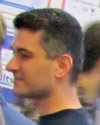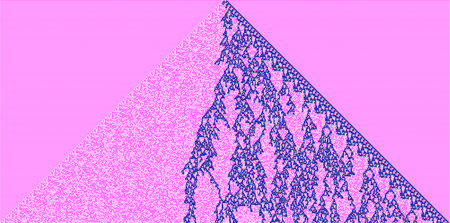#Wolfram Summer School

# Alumni# Melih Ozlem

Summer School

Class of 2010

## Bio

Melih earned his Ph.D. in mathematics. His interests are in the areas of differential equations, numerical analysis, mathematical modeling of complex systems, and computer science.

## Project: NKS on Discretized Partial Differential Equations

We study the parameter space for the PDE u_tt=u_xx+(1-u^2)(1+u) using numerical methods and NKS techniques. The numerical discretization provides a simple set of rules which we use to evolve a given initial state through time. Complex patterns can emerge from simple changes to the initial and boundary conditions, similar to what one may see in a continuous CA simulation. We consider initial conditions of the form u(x,0)=A*exp(-100(x-0.5)^2)+B, u_t(x,0)=0 together with reflective, as well as Dirichlet, boundaries. We further aim to explore the portion of the parameter space for the numerical discretization itself, where one may normally encounter numerical instabilities.

## Favorite Four-Color Totalistic Cellular Automaton

Rule 60177• 21st Annual Wolfram Summer School
• Bentley University, Waltham, MA, USA
• June 25–July 15, 2023